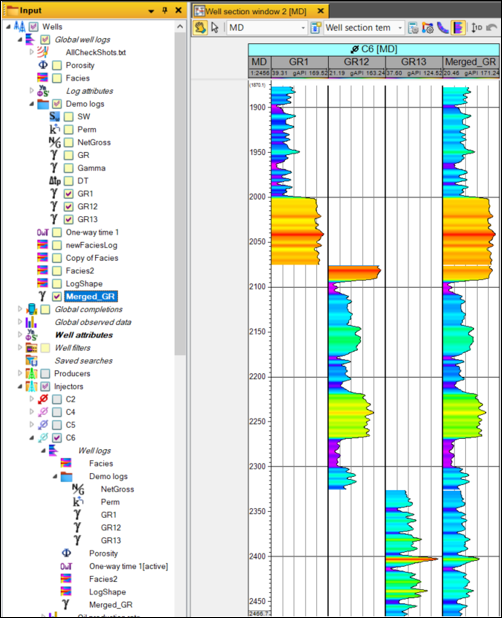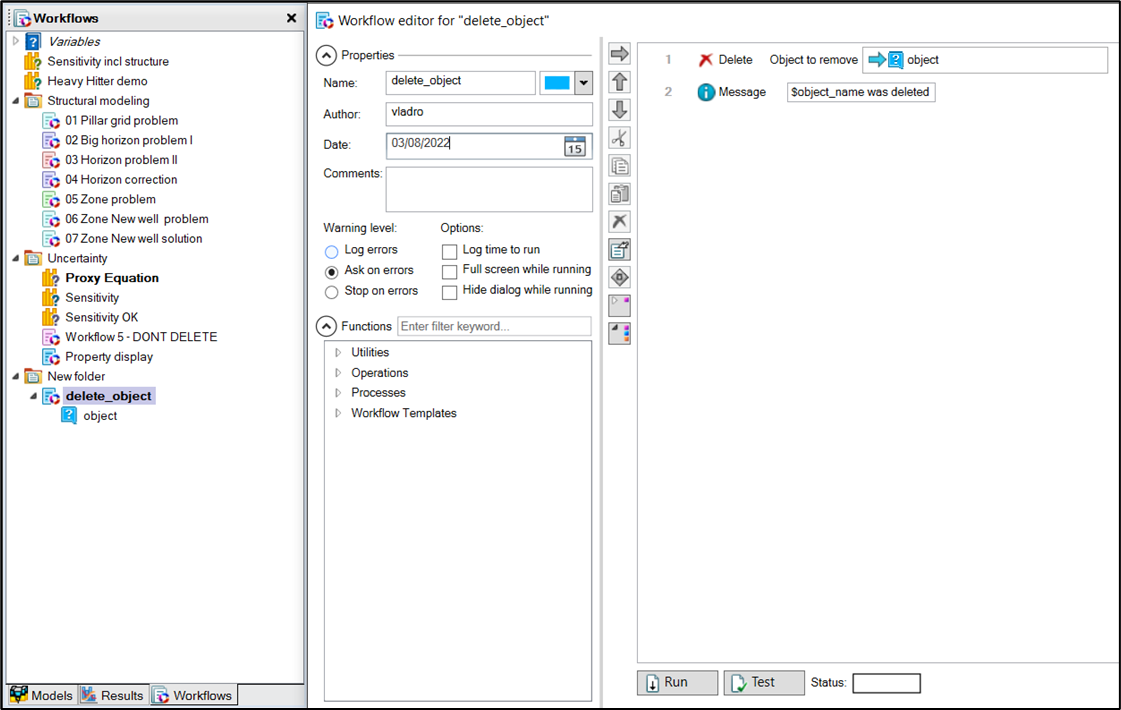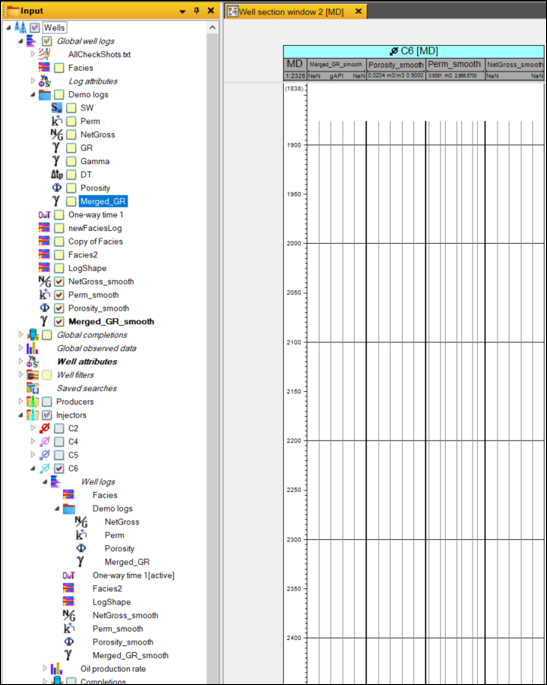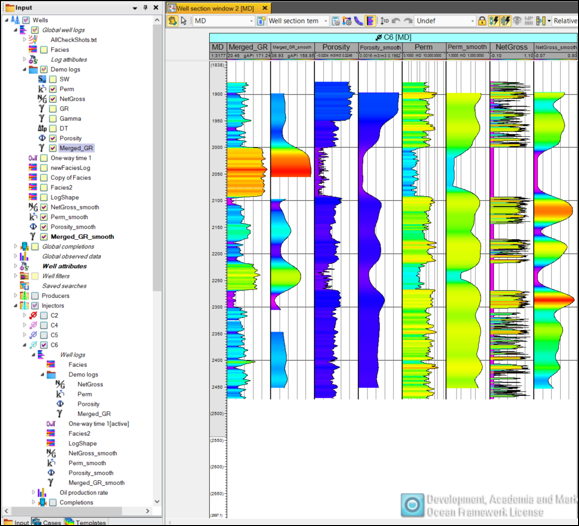# Cegal Prizm Python Tool Pro - Well log operations#

## Enabling data science against Petrel#

In this Notebook, we show how to retrieve well logs from a well, combine multiple logs into a single log, delete logs, apply a smoothing algorithm, and send data back to Petrel

### Python libraries#

For this Notebook to work, additional Python libraries are needed.

:

# This Notebook uses the following packages

import pandas as pd
from matplotlib import pyplot as plt
import ipywidgets as widgets
import os, sys
from random import sample
import scipy

#Packages used to connect to petrel and to access relevant data

from cegalprizm.pythontool import PetrelConnection
from cegalprizm.pythontool.welllog import WellLog, DiscreteWellLog


## Connect to Petrel#

The first step is to establish a connection to Petrel. We will create a Python variable called ‘petrel’ which we will use for accessing the Petrel data.

:

# Make sure you have Petrel open with the project you want to work on and run the following command

petrel = PetrelConnection(allow_experimental=True)

print('Connected to {}'.format(petrel.get_current_project_name()))

Connected to Gullfaks_dataset3.pet


## Select a well and retrieve the well logs#

:

# Method 1: selecting the desired well using a widget

well_names = sorted(list(petrel.wells.keys()))
well_name_widget = widgets.Dropdown(
options=well_names,
value=well_names,
description='Select well:',
disabled=False,
)
display(well_name_widget)

:

# Method 2 : assign a particular well to a variable
well_list=sorted(list(petrel.wells.keys()))
well_C6= well_list
print(well_C6)

Input/Wells/Injectors/C6

:

#Method 1.1: Retrieve well logs using a widget
from random import sample
well = petrel.wells[well_name_widget.value]
w = widgets.SelectMultiple(
options=log_names,
value=sample(log_names, min(len(log_names)//2, 6)),
rows=len(log_names),
description='Select logs',
)

display(w)

:

#Method 2.2: Assign the logs to a variable
print(C6_contLogs)

[WellLog(petrel_name="NetGross"), WellLog(petrel_name="Perm"), WellLog(petrel_name="GR1"), WellLog(petrel_name="GR12"), WellLog(petrel_name="GR13"), WellLog(petrel_name="Porosity"), WellLog(petrel_name="One-way time 1"), WellLog(petrel_name="Merged_GR")]


### Getting all logs interpolated to the same depths in a Pandas DataFrame#

:

logs_df = well.logs_dataframe(C6_contLogs[0:6])
# Sets MD as index
logs_df = logs_df.set_index('MD')
#logs_df = logs_df[list(C6_contLogs[1:5])]
logs_df.loc[2200:2500]

:

NetGross Perm GR1 GR12 GR13 Porosity TWT TVD
MD
2200.222256 0.036268 104.219218 NaN 71.364325 NaN 0.091910 2008.914269 2195.166702
2200.722256 0.532327 116.146416 NaN 73.078747 NaN 0.090269 2009.222128 2195.615601
2201.222256 0.000000 137.262845 NaN 74.734042 NaN 0.088702 2009.529775 2196.064228
2201.722256 0.000000 169.863955 NaN 76.315481 NaN 0.088083 2009.837268 2196.512666
2202.222256 0.746174 199.066936 NaN 76.940353 NaN 0.088649 2010.144549 2196.960839
... ... ... ... ... ... ... ... ...
2471.222256 0.000000 81.100040 NaN NaN 80.895119 0.089047 2162.230573 2423.318593
2471.722256 0.000000 90.980329 NaN NaN 75.967539 NaN 2162.510717 2423.735403
2472.222256 0.000000 NaN NaN NaN NaN NaN 2162.790861 2424.152214
2472.722256 0.000000 NaN NaN NaN NaN NaN 2163.071006 2424.569024
2473.222256 0.000000 NaN NaN NaN NaN NaN 2163.351150 2424.985834

547 rows × 8 columns

## Plotting logs#

:

log_types = logs_df.columns
n = len(log_types)
fig, ax = plt.subplots(1, n-2, figsize=(2*n, 10), sharey = True)

for i in range(n-2):
lt = log_types[i]
ax[i].plot(logs_df[lt], logs_df.index, color='C'+str(i))
ax[i].set_title(lt)
ax[i].grid()

plt.gca().invert_yaxis()## Merging logs#

It looks like our GR-log is split into 3 log tracks. Let’s try and merge all 3 logs into a new GR log:

:

log_types = logs_df.columns
n = len(log_types)
fig, ax = plt.subplots(1, n-5, figsize=(n, 15), sharey = True)

for i in range(n-5):
lt = log_types[i+2]
ax[i].plot(logs_df[lt], logs_df.index, color='C'+str(i))
ax[i].set_title(lt)
ax[i].grid()

plt.gca().invert_yaxis()Let’s create a new column in our DataFrame called merged_GR and use the combine_first() function to combine the three logs:

:

logs_df['merged_GR']=logs_df['GR1'].combine_first(logs_df['GR12'])
logs_df['merged_GR']=logs_df['merged_GR'].combine_first(logs_df['GR13'])
logs_df.loc[2200:2500]

:

NetGross Perm GR1 GR12 GR13 Porosity TWT TVD merged_GR
MD
2200.222256 0.036268 104.219218 NaN 71.364325 NaN 0.091910 2008.914269 2195.166702 71.364325
2200.722256 0.532327 116.146416 NaN 73.078747 NaN 0.090269 2009.222128 2195.615601 73.078747
2201.222256 0.000000 137.262845 NaN 74.734042 NaN 0.088702 2009.529775 2196.064228 74.734042
2201.722256 0.000000 169.863955 NaN 76.315481 NaN 0.088083 2009.837268 2196.512666 76.315481
2202.222256 0.746174 199.066936 NaN 76.940353 NaN 0.088649 2010.144549 2196.960839 76.940353
... ... ... ... ... ... ... ... ... ...
2471.222256 0.000000 81.100040 NaN NaN 80.895119 0.089047 2162.230573 2423.318593 80.895119
2471.722256 0.000000 90.980329 NaN NaN 75.967539 NaN 2162.510717 2423.735403 75.967539
2472.222256 0.000000 NaN NaN NaN NaN NaN 2162.790861 2424.152214 NaN
2472.722256 0.000000 NaN NaN NaN NaN NaN 2163.071006 2424.569024 NaN
2473.222256 0.000000 NaN NaN NaN NaN NaN 2163.351150 2424.985834 NaN

547 rows × 9 columns

We can now display the new merged_GR log:

:

log_types=logs_df.columns[[2,3,4,8]]
n=len(log_types)
fig, ax = plt.subplots(1, n, figsize=(8, 15), sharey = True)

for i in range(n):
lt = log_types[i]
ax[i].plot(logs_df[lt], logs_df.index, color='C'+str(i))
ax[i].set_title(lt)
ax[i].grid()

plt.gca().invert_yaxis()## Writing the result back to Petrel#

We can either overwrite an existing log or create a new log. We can create a new log by cloning the GR1 log (without cloning its values) and then pass the merged_GR values to the cloned log using set_values().

:

# Clone the GR1 log
copy_GR1=C6_contLogs.clone('Merged_GR', copy_values=False)
# Assign the MD values to a variable
MD_index=logs_df.index.to_numpy()
# Assign the merged_GR values to a variable
MergedGR_values=logs_df['merged_GR'].to_numpy()
#Assign the MD and Merged_GR values to the new log
copy_GR1.set_values(MD_index,MergedGR_values)


The image below shows the 3 GR logs and the merged_GR logs in Petrel:## Delete logs#

Let’s select the 3 incomplete GR logs and delete them from the project. We can select the 3 GR logs using a widget:

:

#Method 1.1: Retrieve well logs using a widget
from random import sample
well = petrel.wells[well_name_widget.value]
w = widgets.SelectMultiple(
options=log_names,
value=sample(log_names, min(len(log_names)//2, 6)),
rows=len(log_names),
description='Select logs',
)

display(w)


To delete the logs, we can use a Petrel Workflow. First, we assign all the workflows in our project to the workflows variable. Then, using list slicing, we select the right workflow. Using a for loop we can pass each of the three logs as the input variable in our workflow and delete them. The image below shows how the workflow looks in Petrel::

workflows = [workflow for workflow in petrel.workflows]
delete_workflow = workflows
delete_workflow_input_variable = delete_workflow.input['object']
delete_workflow.run(args={delete_workflow_input_variable:petrel.global_well_logs['Input/Wells/Global well logs/Demo logs/'+w.value[log]]})


We can check the available logs in our well and make sure the logs are deleted:

:

C6_contLogs=[log for log in well.logs if type(log) is WellLog]
print (log.petrel_name)

NetGross
Perm
Porosity
One-way time 1
Merged_GR


## Smoothing logs#

To smooth well logs, we can use a moving window gaussian filter on each column in the DataFrame.

:

# update the df
logs_df = well.logs_dataframe(C6_contLogs)
# Sets MD as index
logs_df = logs_df.set_index('MD')

# Apply a moving window gaussian filter on each variable in the DataFrame.
smooth_logs_df = logs_df.rolling(80, win_type = 'gaussian', center = True).mean(std = 20)

#Plot the smoothed logs on top of the original logs
log_types=logs_df.columns[[0,1,2,4]]
n = len(log_types)
fig, ax = plt.subplots(1, n, figsize=(3*n, 10), sharey = True)

for i in range(n):
lt = log_types[i]
ax[i].plot(logs_df[lt], logs_df.index, color='C'+str(i), label = 'raw')
ax[i].plot(smooth_logs_df[lt], smooth_logs_df.index, color='k', linewidth = 2, label = 'smoothed')
ax[i].set_title(lt)
ax[i].grid()
ax[i].legend()

plt.gca().invert_yaxis()Let’s clone each log and create an empty copy in Petrel:

:

for i in range(n):
old_log_path = well_name_widget.value + '/Well logs/Demo logs/' + log_types[i]
old_log = petrel.well_logs[old_log_path]
try:
new_sm_log = old_log.clone(log_types[i]+'_smooth')  # create the clone if it doesn't already exist
except Exception:
new_sm_log = petrel.well_logs[old_log_path+'_smooth'] # get if it already exists
print(old_log)
print(new_sm_log)

WellLog(petrel_name="NetGross")
WellLog(petrel_name="NetGross_smooth")
WellLog(petrel_name="Perm")
WellLog(petrel_name="Perm_smooth")
WellLog(petrel_name="Porosity")
WellLog(petrel_name="Porosity_smooth")
WellLog(petrel_name="Merged_GR")
WellLog(petrel_name="Merged_GR_smooth")


We have now created 4 new logs in Petrel:### Insert values into well logs#

We can now write some values to our cloned logs:

:

for i in range(n):
md = smooth_logs_df.index.to_numpy()
new_sm_values = smooth_logs_df[log_types[i]].to_numpy()
old_log_path = well_name_widget.value + '/Well logs/' + log_types[i]
old_log = petrel.well_logs[old_log_path+'_smooth']
new_sm_log = old_log